# Leg and height

Solve right triangle with height v = 9.6 m and shorter cathetus b = 17.3 m.

a =  11.54 m
b =  17.3 m
c =  20.8 m

### Step-by-step explanation:

Try calculation via our triangle calculator.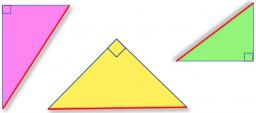Did you find an error or inaccuracy? Feel free to write us. Thank you!Tips to related online calculators
Do you have a linear equation or system of equations and looking for its solution? Or do you have a quadratic equation?
Pythagorean theorem is the base for the right triangle calculator.

#### You need to know the following knowledge to solve this word math problem:

We encourage you to watch this tutorial video on this math problem:

## Related math problems and questions:

• Sides of right angled triangleOne leg is 1 m shorter than the hypotenuse, and the second leg is 2 m shorter than the hypotenuse. Find the lengths of all sides of the right-angled triangle.
• TriangleCalculate the triangle sides if its area S = 630 and the second cathetus is shorter by 17.
• Leg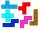Determine the trapezoid area with bases 71 and 42, and the height is 4 shorter than its leg.
• Euklid4The legs of a right triangle have dimensions 244 m and 246 m. Calculate the length of the hypotenuse and the height of this right triangle.
• R triangleCalculate the right triangle area whose longer leg is 6 dm shorter than the hypotenuse and 3 dm longer than the shorter leg.
• Triangle KLMIn the rectangular triangle KLM, where is hypotenuse m (sketch it!). Find the length of the leg k and the height of triangle h if the hypotenuse's segments are known MK = 5cm and ml = 15 cm.
• RT perimeterThe leg of the rectangular triangle is 7 cm shorter than the second leg and 8 cm shorter than the hypotenuse. Calculate the triangle circumference.
• Right trapezoidThe right trapezoid has bases 3.2 cm and 62 mm long. The shorter leg has a length of 0.25 dm. Calculate the lengths of the diagonals and the second leg.
• Without Euclid lawsRight triangle ABC with right angle at the C has a=14 and hypotenuse c=26. Calculate the height h of this triangle without the use of Euclidean laws.
• Euclid theoremsCalculate the sides of a right triangle if leg a = 6 cm, and a section of the hypotenuse, which is located adjacent to the second leg b is 5cm.
• Euclid3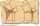Calculate height and sides of the right triangle, if one leg is a = 81 cm and section of hypotenuse adjacent to the second leg cb = 39 cm.
• Triangle ABCRight triangle ABC with right angle at the C, |BC|=18, |AB|=33. Calculate the height of the triangle hAB to the side AB.
• One leg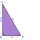One leg of a right triangle is 1 feet longer than the other leg. The hypotenuse is 5 feet. Find the lengths of the three sides of the triangle.
• RT - hypotenuse and altitudeRight triangle BTG has hypotenuse g=117 m and altitude to g is 54 m. How long are hypotenuse segments?
• TentCalculate how many liters of air will fit in the tent that has a shield in the shape of an isosceles right triangle with legs r = 3 m long the height = 1.5 m and a side length d = 5 m.
• Right triangle - leg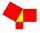Calculate to the nearest tenth cm leg length in the right-angled triangle with hypotenuse length 9 cm and 7 cm long leg.
• The ditchDitch with a cross-section of an isosceles trapezoid with bases 2m and 6m are deep 1.5m. How long is the slope of the ditch?